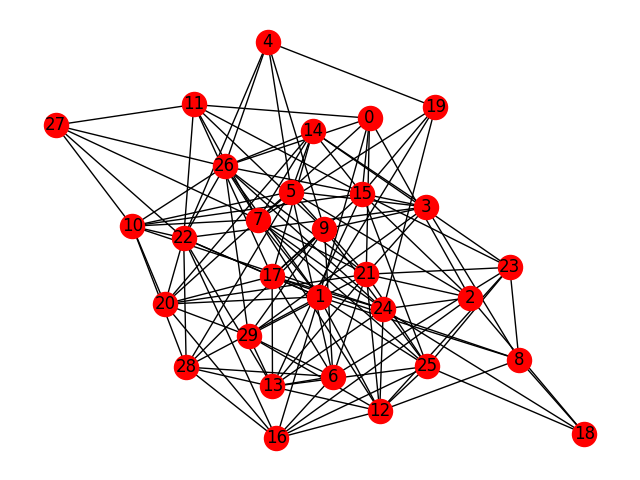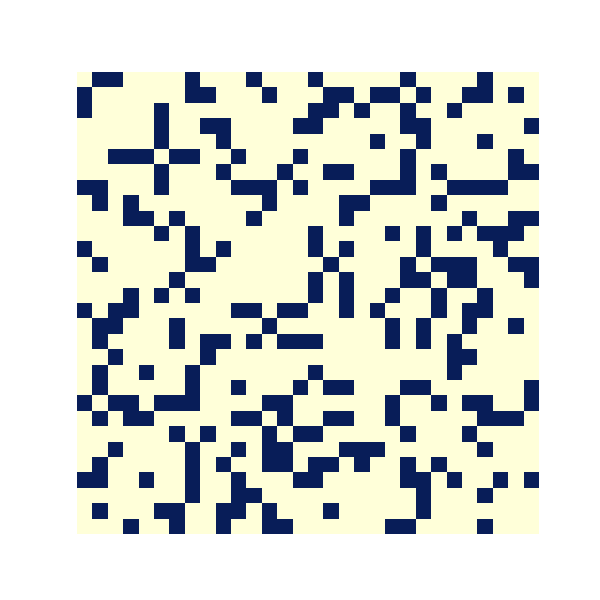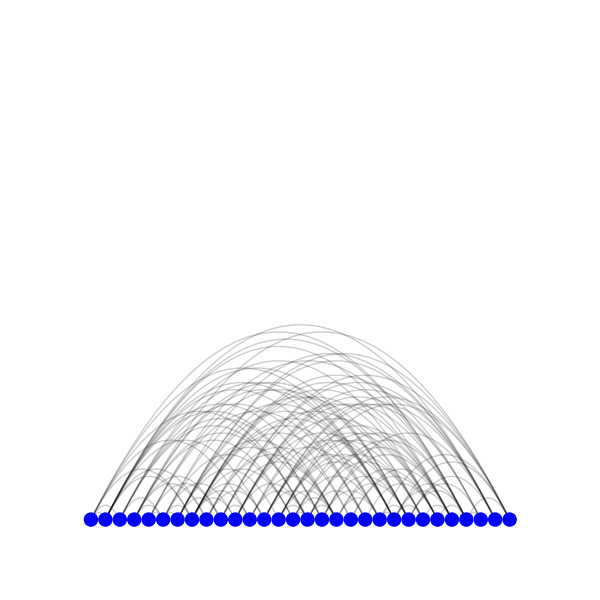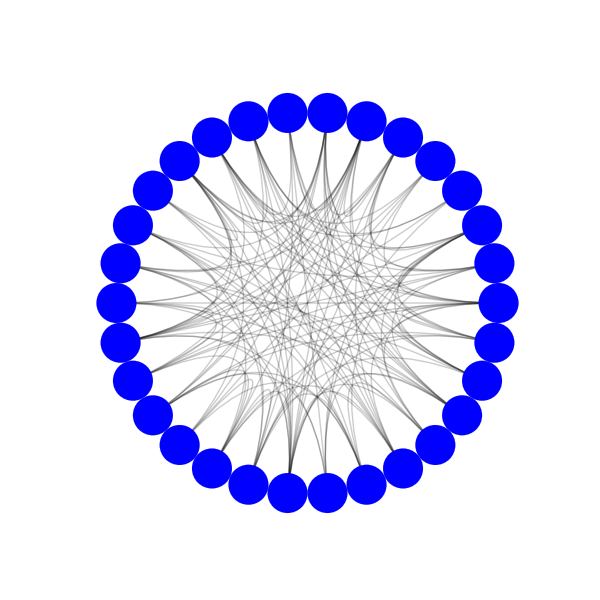# Usage¶

## Plots¶

All nxviz plot objects take in a Networkx graph object and output a plot object. The following examples will use a randomly generated Networkx graph. The following code uses Networkx’s built-in drawing function.

```import networkx as nx
import matplotlib.pyplot as plt

er = nx.erdos_renyi_graph(30, 0.3)
nx.draw(er)
plt.show()
```Networkx built-in draw function

### Matrix Plots¶

```from nxviz.plots import MatrixPlot

m = MatrixPlot(er)
m.draw()
plt.show()
```nxviz matrix plot

### Arc Plots¶

```from nxviz.plots import ArcPlot

a = ArcPlot(er)
a.draw()
plt.show()
```nxviz arc plot

### Circos Plots¶

```from nxviz.plots import CircosPlot

c = CircosPlot(er)
c.draw()
plt.show()
```nxviz Circos plot

For a full list of parameters, see the `nxviz.plots.CircosPlot` API documentation.

The above is a basic overview of the available plots. For more detailed examples of these plots in action, please see the examples here.